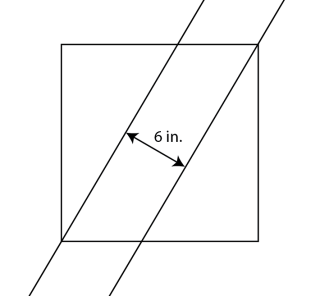# Square Thirds

From Problem of the Fortnight, CS at San Diego State University.Fig. 1: The parallel lines divide the square into three parts of equal area
A square is cut into three equal area regions by two parallel lines that are 6 inches apart, each one passing through exactly one of two diagonally opposed vertices. What is the area of the square?